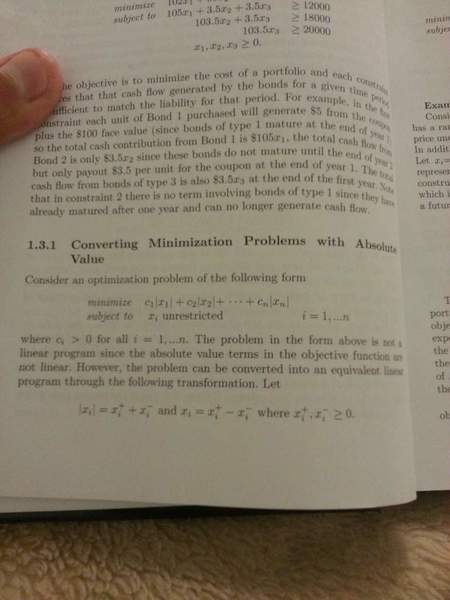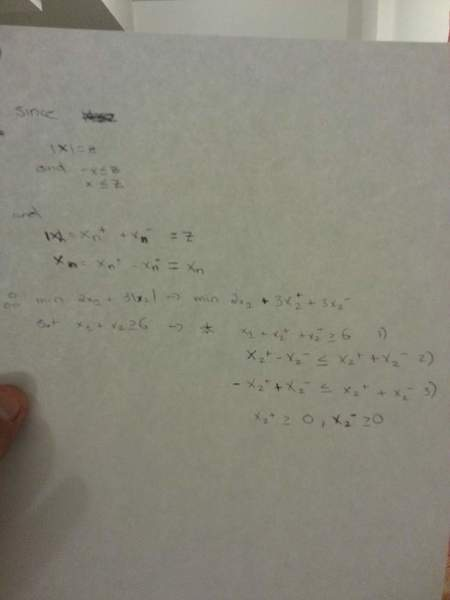# Converting a linear optimization problem with absolute values

## Homework Statement

Here is an alternative approach to handling absolute value terms as the decision variables: abs(x) is the smallest value z that satisfies x $\leq$ z and -x $\leq$ z. Using this,convert the following into a lp

Min 2x1 + 3abs(x2)

S.T x1 + x2 $\geq$ 6

## Homework Equations

Here is a pretext from my textbook that shows how to do it a different way## The Attempt at a Solution

After a while of staring at it ,I gave it a tryWhat do you guys think?

Last edited by a moderator:

Ray Vickson
Homework Helper
Dearly Missed

## Homework Statement

Here is an alternative approach to handling absolute value terms as the decision variables: abs(x) is the smallest value z that satisfies x $\leq$ z and -x $\leq$ z. Using this,convert the following into a lp

Min 2x1 + 3abs(x2)

S.T x1 + x3 $\geq$ 6

## Homework Equations

Here is a pretext from my textbook that shows how to do it a different way

View attachment 61918

## The Attempt at a Solution

After a while of staring at it ,I gave it a try

View attachment 61920

What do you guys think?

I think there is something wrong with the problem statement: it has ##x_1## and ##x_2## in the objective but ##x_1## and ##x_3## in the constraint.
Mod note: This is now fixed.
As written, the problem does not have a finite solution, since we can have ##x_1 \rightarrow -\infty##, ##x_3 = 6 - x_1 \rightarrow +\infty## and ##x_2 = 0##. If you really intend to have ##x_1 \geq 0## you need to say so.

In any case, you need only one constraint, not three. Basically, it is one of the standard methods for such problems to just replace ##x_2## by ##x_2^+ - x_2^-## (with ##x_2^+, x_2^- \geq 0##) and to write ##x_2^+ + x_2^-## in place of ##|x_2|## in the objective.

Last edited by a moderator:
Hey

Ya sorry I just noticed as well that I made a mistake. Theres no x3,I meant x2.

Anyways , ya the way you have said I already know how to do. But the reason im confused is that the question asks that the aforementioned observation be used in answering the question.

P.S I cant seem to edit the question to fix the mistake

Mark44
Mentor
Hey

Ya sorry I just noticed as well that I made a mistake. Theres no x3,I meant x2.

Anyways , ya the way you have said I already know how to do. But the reason im confused is that the question asks that the aforementioned observation be used in answering the question.

P.S I cant seem to edit the question to fix the mistake
I fixed it for you. Members have only a few hours to go back and edit their posts, but mentors don't have that restriction.

•1 person
Ray Vickson
Homework Helper
Dearly Missed
Hey

Ya sorry I just noticed as well that I made a mistake. Theres no x3,I meant x2.

Anyways , ya the way you have said I already know how to do. But the reason im confused is that the question asks that the aforementioned observation be used in answering the question.

P.S I cant seem to edit the question to fix the mistake

There are two *different* ways of handling such problems, and you have mixed them up. For problems in which |x| appears only in the objective, and with a coefficient c > 0, we can:
(1) Replace ##x## in the constraints by ##x^+ - x^-## and ##|x|## by ##x^+ + x^-## in the objective; or
(2) keep x unchanged in the constraints, replace |x| in the objective by a new variable z, and add two constraints z ≥ x and z ≥ -x.

•1 person
There are two *different* ways of handling such problems, and you have mixed them up. For problems in which |x| appears only in the objective, and with a coefficient c > 0, we can:
(1) Replace ##x## in the constraints by ##x^+ - x^-## and ##|x|## by ##x^+ + x^-## in the objective; or
(2) keep x unchanged in the constraints, replace |x| in the objective by a new variable z, and add two constraints z ≥ x and z ≥ -x.

Ya i know the first way as its stated in the textbook but the way the question is being asked to be done is how you stated in (2).

So if I replace |x2| in this case by z and add the two constraints like you said, what i am wondering is what do the x2 and -x2 mean in the constraints because they are not in the objective function

Min 2x1 +3z

S.T x1 + x2 ≥ 6
z ≥ x2 and z ≥ -x2

Ray Vickson
Homework Helper
Dearly Missed
Ya i know the first way as its stated in the textbook but the way the question is being asked to be done is how you stated in (2).

So if I replace |x2| in this case by z and add the two constraints like you said, what i am wondering is what do the x2 and -x2 mean in the constraints because they are not in the objective function

Min 2x1 +3z

S.T x1 + x2 ≥ 6
z ≥ x2 and z ≥ -x2

Stop worrying; your formulation is OK exactly as it stands. When you try to make z small, that will restrict x_2 in the constraints, so everything *really is* connected together. If you don't believe it, try solving both formulations (either by hand or by using an LP computer package).

•1 person